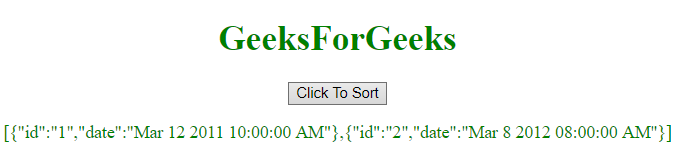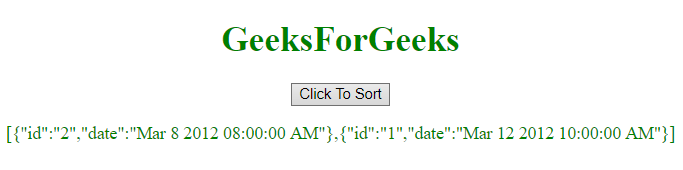Related Articles
Sort an Object Array by Date in JavaScript
• Last Updated : 07 May, 2019

To sort an array of object by date, there is a number of methods but we’re going to see few of the most preferred methods.

Date Object: The Date object in JavaScript is used to represent a moment of time. This time value is since 1 January 1970 UTC (Coordinated Universal Time). We can create date using the Date object by calling the new Date() constructor as shown in the below syntax.
Syntax:

```new Date();
new Date(value);
new Date(dateString);
new Date(year, month, day, hours, minutes, seconds, milliseconds);
```

Parameters:

• value: This value is the number of milliseconds since January 1, 1970, 00:00:00 UTC.
• dateString: It represents a date format.
• year: It represents the integer values which ranges from years 1900 to 1999.
• month: It is represented by integer values which ranges from 0 for January to 11 for December.
• day: It is an optional parameter. It is represented by integer value for the day of the month.
• hours: It is optional parameter. It is represented by an integer value for the hour of the day.
• minutes: It is optional parameter. It is represented by an integer value for the minute of a time.
• seconds: It is optional parameter. It is represented by integer value for the second of a time.
• milliseconds: It is optional parameter. It is represented by integer value for the millisecond of a time.

Example 1: This example sorts the array of objects by date by using Date object.

 ` ``<``html``> ``    ``<``head``> ``        ``<``title``> ``            ``JavaScript | Sort Object Array By Date``        `` ``    ````     ` `    ``<``body` `style` `= ``"text-align:center;"``> ``         ` `        ``<``h1` `style` `= ``"color:green;"` `> ``            ``GeeksForGeeks ``        `` ``         ` `        ``<``button` `onclick` `= ``"geeks_outer()"``> ``            ``Click To Sort ``        `` ``     ` `        ``<``p` `id` `= ``"GFG_P"` `style` `= ``"color:green;"``>``         ` `        ``<``script``> ``            ``var array = [{id: "1", date: "Mar 11 2012 10:00:00 AM"},``                        ``{id: "2", date: "Mar 8 2012 08:00:00 AM"}];``             ` `            ``var el = document.getElementById("GFG_P");``            ``function geeks_outer() {``                ``array.sort(function(a, b){``     ` `                    ``return new Date(a.date) - new Date(b.date);``                ``});``             ` `                ``el.innerHTML = JSON.stringify(array);``            ``}``             ` `        `` ``    `` ``                    `

Output:

• Before clicking on the button:• After clicking on the button:Example 2: This example is same as previous one but with a little modification in the sort function.

 ` ``<``html``> ``    ``<``head``> ``        ``<``title``> ``            ``JavaScript | Sort Object Array By Date. ``        `` ``    ````     ` `    ``<``body` `style` `= ``"text-align:center;"``> ``         ` `        ``<``h1` `style` `= ``"color:green;"` `> ``            ``GeeksForGeeks ``        `` ``         ` `        ``<``button` `onclick` `= ``"geeks_outer()"``> ``            ``Click To Sort``        `` ``         ` `        ``<``p` `id` `= ``"GFG_P"` `style` `= ``"color:green;"``>``         ` `        ````        ``<``script``> ``            ``var array = [{id: "1", date: "Mar 12 2012 10:00:00 AM"},``                        ``{id: "2", date: "Mar 8 2012 08:00:00 AM"}];``             ` `            ``var el = document.getElementById("GFG_P");``         ` `            ``function geeks_outer() {``                ``array.sort(GFG_sortFunction);``                ``el.innerHTML = JSON.stringify(array);``            ``}``             ` `            ``function GFG_sortFunction(a, b) { ``                ``var dateA = new Date(a.date).getTime();``                ``var dateB = new Date(b.date).getTime();``                ``return dateA > dateB ? 1 : -1; ``            ``}; ``        `` ``    `` ``                    `

Output:

• Before clicking on the button:• After clicking on the button:My Personal Notes arrow_drop_up